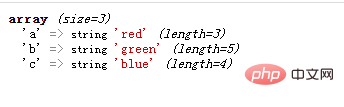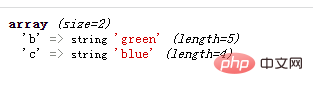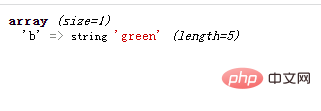# php中两个数组求交集的函数有哪些php中提供例如多个求数组交集的函数：

• array_intersect()：比较数组，返回两个数组的交集（只比较键值）。

• array_intersect_assoc()：比较数组，返回两个数组的交集（比较键名和键值）。

• array_intersect_key()：比较数组，返回两个数组的交集（只比较键名）。

• array_intersect_uassoc()：比较数组，返回两个数组的交集（比较键名和键值，使用用户自定义比较函数）。

• array_intersect_ukey()：比较数组，返回两个数组的交集（只比较键名，使用用户自定义比较函数）。

• array_uintersect()：比较数组，返回两个数组的交集（只比较键值，使用一个用户自定义比较函数）。

• array_uintersect_assoc()：比较数组，返回两个数组的交集（比较键名和键值，使用内建函数比较，使用用户自定义函数比较键值）。

• array_uintersect_uassoc()：比较数组，返回两个数组的交集（比较键名和键值，使用两个用户自定义的比较函数）。

1、array_intersect()函数

array_intersect() 函数用于比较两个（或更多个）数组的值，并返回交集。

```<?php
\$a1=array("a"=>"red","b"=>"green","c"=>"blue","d"=>"yellow");
\$a2=array("e"=>"red","f"=>"green","g"=>"blue");

\$result=array_intersect(\$a1,\$a2);
var_dump(\$result);
?>```2、array_intersect_assoc()函数

array_intersect_assoc() 函数用于比较两个（或更多个）数组的键名和键值，并返回交集。

```<?php
\$a1=array("a"=>"red","b"=>"green","c"=>"blue","d"=>"yellow");
\$a2=array("a"=>"red","b"=>"green","c"=>"blue");

\$result=array_intersect_assoc(\$a1,\$a2);
var_dump(\$result);
?>```3、array_intersect_key()函数

```<?php
\$a1=array("a"=>"red","b"=>"green","c"=>"blue");
\$a2=array("a"=>"red","c"=>"blue","d"=>"pink");

\$result=array_intersect_key(\$a1,\$a2);
var_dump(\$result);
?>```• array_intersect_uassoc()

• array_intersect_ukey()

• array_uintersect()

• array_uintersect_assoc()

• array_uintersect_uassoc()

```<?php
function myfunction(\$a,\$b)
{
if (\$a===\$b)
{
return 0;
}
return (\$a>\$b)?1:-1;
}

\$a1=array("a"=>"red","b"=>"green","c"=>"blue");
\$a2=array("d"=>"red","b"=>"green","e"=>"blue");

\$result=array_intersect_uassoc(\$a1,\$a2,"myfunction");
var_dump(\$result);
?>```1/1